# Fréchet derivative

(diff) ← Older revision | Latest revision (diff) | Newer revision → (diff)

strong derivative

The most widespread (together with the Gâteaux derivative, which is sometimes called the weak derivative) derivative of a functional or a mapping. The Fréchet derivative of a mappingof a normed spaceinto a normed spaceat a pointis the linear continuous operator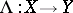satisfying the condition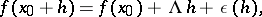where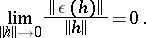The operator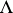satisfying these conditions is unique (if it exists) and is denoted by; the linear mappingis called the Fréchet differential. Ifhas a Fréchet derivative at, it is said to be Fréchet differentiable. The most important theorems of differential calculus hold for Fréchet derivatives — the theorem on the differentiation of a composite function and the mean value theorem. Ifis continuously Fréchet differentiable in a neighbourhood of a pointand if the Fréchet derivative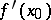atis a homeomorphism of the Banach spacesand, then the inverse mapping theorem holds. See also Differentiation of a mapping.